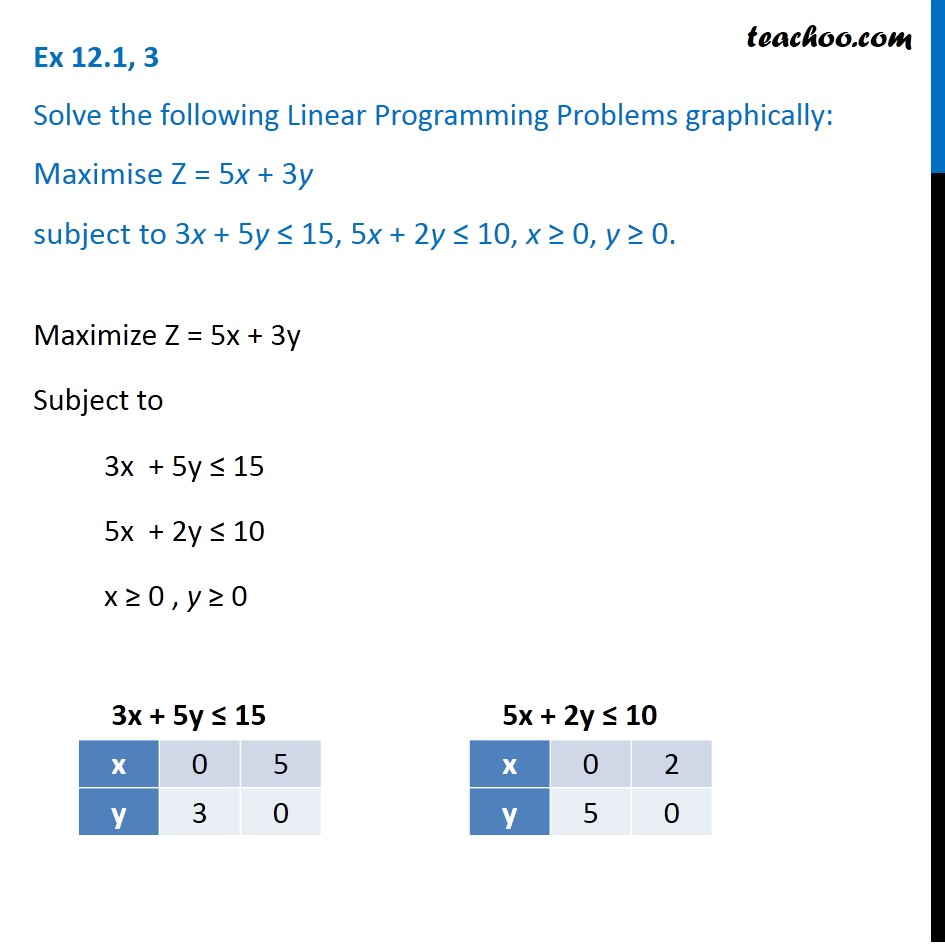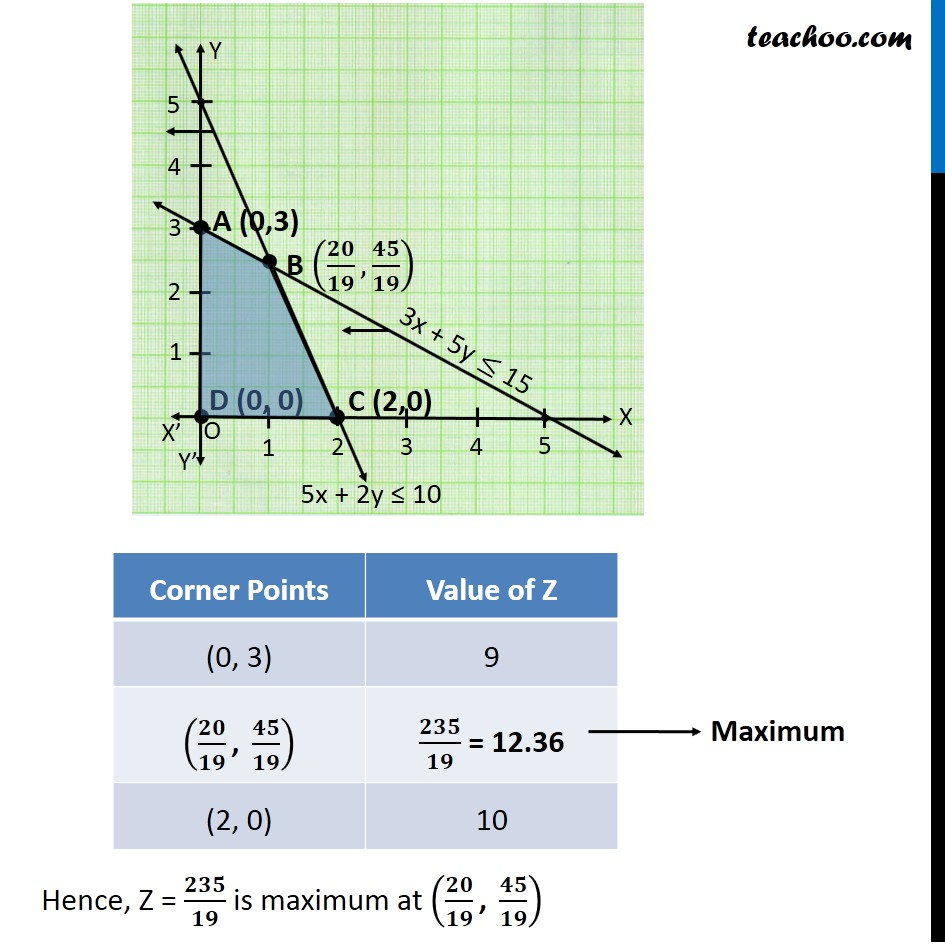Ex 12.1

Chapter 12 Class 12 Linear Programming
Serial order wiseLearn in your speed, with individual attention - Teachoo Maths 1-on-1 Class

### Transcript

Ex 12.1, 3 Solve the following Linear Programming Problems graphically: Maximise Z = 5x + 3y subject to 3x + 5y ≤ 15, 5x + 2y ≤ 10, x ≥ 0, y ≥ 0. Maximize Z = 5x + 3y Subject to 3x + 5y ≤ 15 5x + 2y ≤ 10 x ≥ 0 , y ≥ 0 Hence, Z = 𝟐𝟑𝟓/𝟏𝟗 is maximum at (𝟐𝟎/𝟏𝟗 ", " 𝟒𝟓/𝟏𝟗)